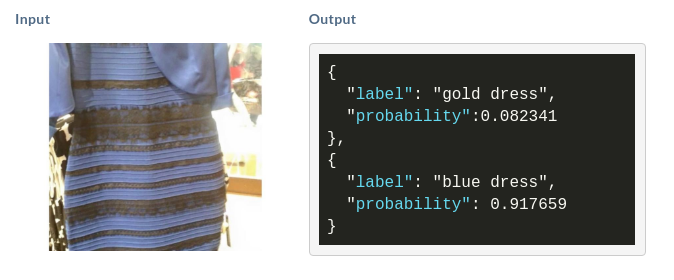# NanoNets：数据不足时如何深度学习

 - VGGNet DeepVideo GNMT 用途 识别图像并分类 识别视频并分类 翻译 输入 图像 视频 英语文本 输出 100 种类别 47 种类别 法语文本 参数数量 1.4 亿个 约 1 亿个 3.8 亿个 数据规模 120 万张已分类图片 110 万个已分类视频 6 百万语句对，3.4 亿个单词 数据集 ILSVRC-2012 Sports-1M WMT'14

## 神经网络（即深度学习）是一种分层式结构，但又能堆叠在一起（就像乐高积木）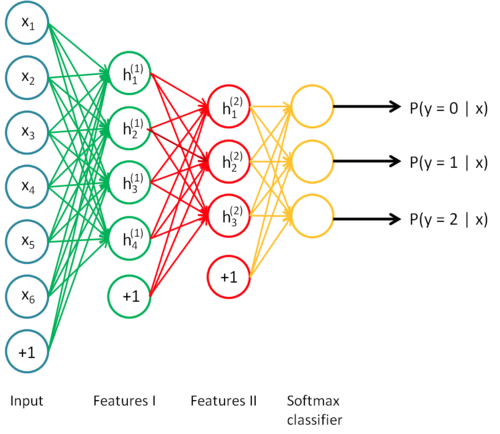## 规模（模型） ∝ 规模（数据） ∝ 复杂度（问题）

(点击放大图像)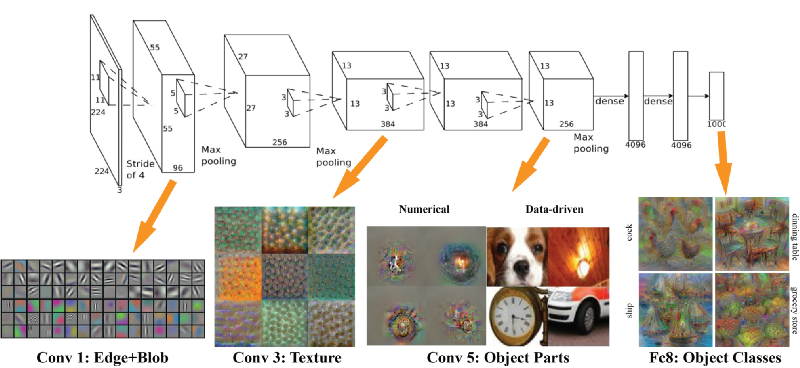AlexNet 在每个环节“看到”的内容

## 迁移学习来搭救！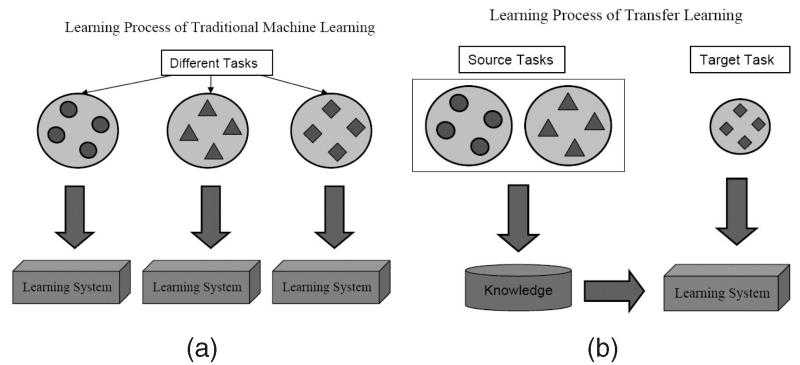Qiang Yang、Sinno Jialin Pan，“A Survey on Transfer Learning”，IEEE Transactions on Knowledge & Data Engineering，vol. 22, no. , pp. 1345–1359, October 2010, doi:10.1109/TKDE.2009.191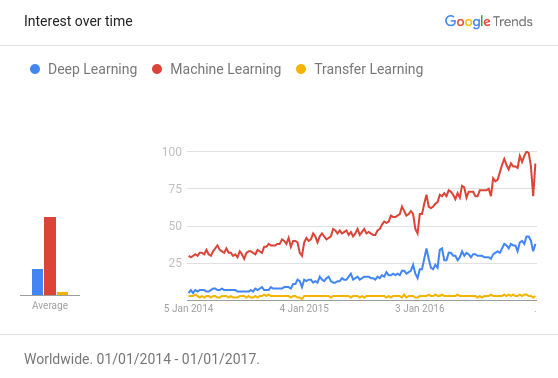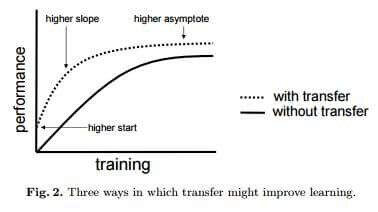## 迁移学习可减小数据量

= [2048+1]*[1+1]~ 4098 个参数

## 迁移学习循序渐进指南?—? 使用示例进行情绪分析

1. 62 篇不包含明确的情绪，将用于对模型进行预训练
2. 8 篇包含明确的情绪，将用于对模型进行训练
3. 2 篇包含明确的情绪，将用于对模型进行测试

### 第 1 步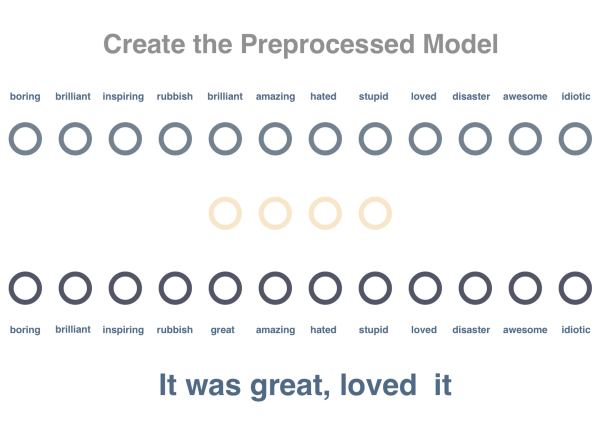graph = tf.Graph()
with graph.as_default():
train_inputs = tf.placeholder(tf.int32, shape=[batch_size])
train_labels = tf.placeholder(tf.int32, shape=[batch_size, 1])
valid_dataset = tf.constant(valid_examples, dtype=tf.int32)
with tf.device('/cpu:0'):
embeddings = tf.Variable(tf.random_uniform([vocabulary_size, embedding_size], -1.0, 1.0))
embed = tf.nn.embedding_lookup(embeddings, train_inputs)
nce_weights = tf.Variable(tf.truncated_normal([vocabulary_size, embedding_size], stddev=1.0 / math.sqrt(embedding_size)))
nce_biases = tf.Variable(tf.zeros([vocabulary_size]))
loss = tf.reduce_mean(tf.nn.nce_loss(nce_weights, nce_biases, embed, train_labels, num_sampled, vocabulary_size))
norm = tf.sqrt(tf.reduce_sum(tf.square(embeddings), 1, keep_dims=True))
normalized_embeddings = embeddings / norm
valid_embeddings = tf.nn.embedding_lookup(normalized_embeddings, valid_dataset)
similarity = tf.matmul(valid_embeddings, normalized_embeddings, transpose_b=True)
init = tf.global_variables_initializer()

pretraining_model.py 托管于 GitHub，查看源文件

### 第 2 步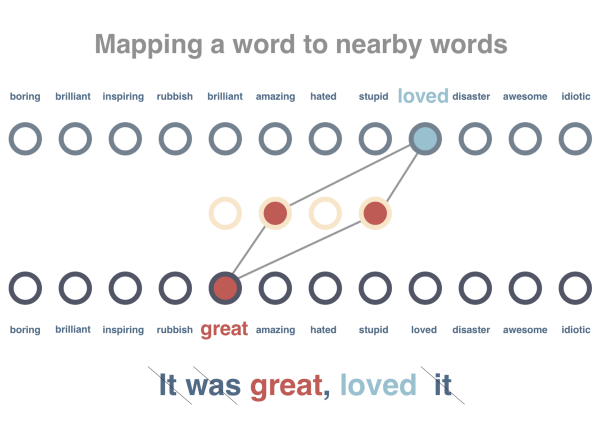with tf.Session(graph=graph) as session:
init.run()
average_loss = 0
for step in range(10001):
batch_inputs, batch_labels = generate_batch(batch_size, num_skips, skip_window)
feed_dict = {train_inputs: batch_inputs, train_labels: batch_labels}
_, loss_val, normalized_embeddings_np = session.run([optimizer, loss, normalized_embeddings], feed_dict=feed_dict)
average_loss += loss_val
final_embeddings = normalized_embeddings.eval()

training_the_pretrained_model.py 托管于 GitHub，查看源文件

### 第 3 步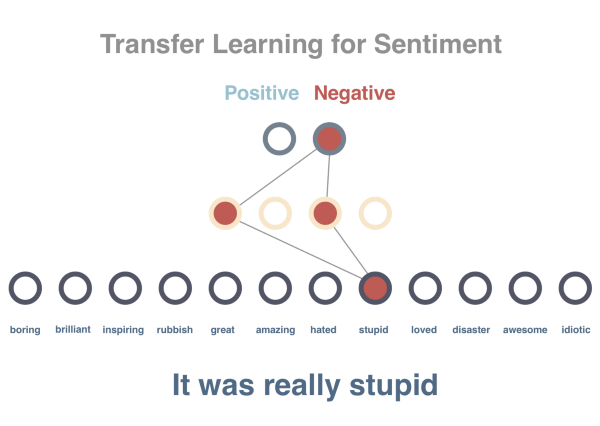input = tf.placeholder("float", shape=[None, x_size])
y = tf.placeholder("float", shape=[None, y_size])
w_1 = tf.Variable(tf.random_normal((x_size, h_size), stddev=0.1))
w_2 = tf.Variable(tf.random_normal((h_size, y_size), stddev=0.1))
h = tf.nn.sigmoid(tf.matmul(X, w_1))
yhat = tf.matmul(h, w_2)
predict = tf.argmax(yhat, dimension=1)
cost    = tf.reduce_mean(tf.nn.softmax_cross_entropy_with_logits(yhat, y))
sess = tf.InteractiveSession()
init = tf.initialize_all_variables()
sess.run(init)

for epoch in range(1000):
for i in range(len(train_X)):
sess.run(updates, feed_dict={X: train_X[i: i + 1], y: train_y[i: i + 1]})
train_accuracy = numpy.mean(numpy.argmax(train_y, axis=1) == sess.run(predict, feed_dict={X: train_X, y: train_y}))
test_accuracy  = numpy.mean(numpy.argmax(test_y, axis=1) == sess.run(predict, feed_dict={X: test_X, y: test_y}))
print("Epoch = %d, train accuracy=%.2f%%, test accuracy=%.2f%%" % (epoch+1,100.*train_accuracy,100.* test_accuracy))

training_the_sentiment_model.py 托管于 GitHub，查看源文件

https://gist.github.com/prats226/9fffe8ba08e378e3d027610921c51a78

## 迁移学习实现过程中的难点

1. 找到预训练所需的大规模数据集
2. 决定用来预训练的模型
3. 两种模型中任何一种无法按照预期工作都将比较难以调试
4. 不确定为了训练模型还需要额外准备多少数据
5. 使用预训练模型时难以决定在哪里停止
6. 在预训练模型的基础上，确定模型所需层和参数的数量
7. 托管并提供组合后的模型
8. 当出现更多数据或更好的技术后，对预训练模型进行更新

## NanoNets 让迁移学习变得更简单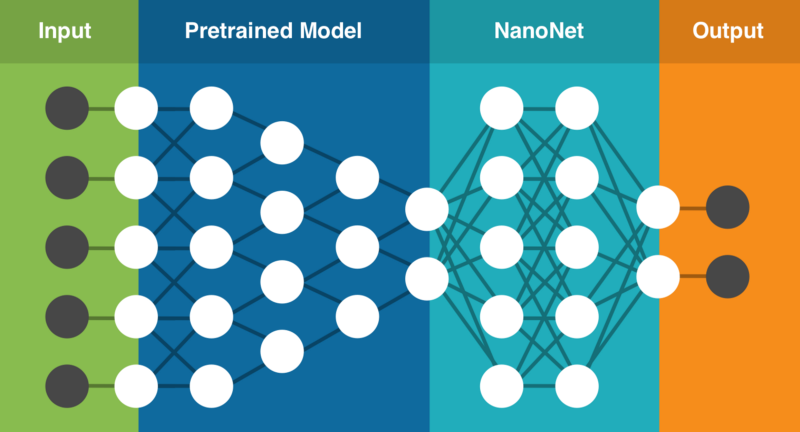NanoNets 的迁移学习技术（该架构仅为基本呈现）

## 构建你的首个 NanoNet（图像分类）

1. 在这里选择你要处理的分类。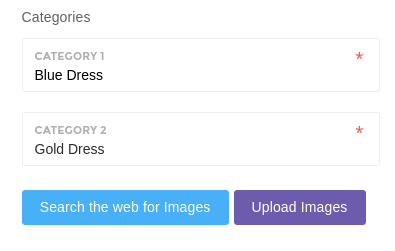2. 一键点击开始搜索网络并构建模型（你也可以上传自己的图片）。3. 解决蓝金裙子的争议（模型就绪后我们会通过简单易用的 Web 界面让你上传测试图片，同时还提供了不依赖特定语言的 API）。